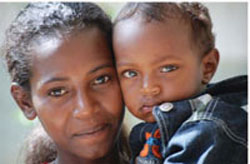# 10.2.2  How to measure dropout rates

The dropout rate is found by comparing the number of infants who start the immunization schedule with the number who complete it. Two measures of dropout rate are routinely used:

In this section, we refer to pentavalent vaccine, which is also known as DPT-HepB-Hib vaccine.

• the dropout rate between infants receiving the first dose of pentavalent vaccine (Penta1) and the third dose (Penta3)
• the dropout rate between receiving the first dose of pentavalent vaccine (Penta1) and the single dose of measles vaccine.

## Pentavalent 1 to pentavalent 3 dropout rate

• What should the interval be between receiving pentavalent 1 and pentavalent 3 vaccines?

• The interval should be 8 weeks: pentavalent 1 is given at 6 weeks of age, and pentavalent 3 is given at 14 weeks.

If an infant fails to complete the schedule of three doses of pentavalent vaccine, it indicates that there is an access problem for the parents, i.e. they have difficulty in getting to (accessing) the immunization sessions for the second or third doses.

The pentavalent 1 to pentavalent 3 dropout rate is calculated using the following equation:

Brackets in an equation mean that you should calculate the answer to whatever is inside the brackets first.

Penta1 to Penta3 dropout rate = (Penta1 – Penta3) ÷ Penta1 x 100%, where:

• Penta1 is the number (or percentage) receiving the first pentavalent vaccine dose
• Penta3 is the number (or percentage) receiving the third dose.
• Imagine that 156 infants received the first dose of pentavalent vaccine at your Health Post last year. The number brought back for the second dose was 140 and the number who received the third dose was 132. What was the Penta1 to Penta3 dropout rate for your Health Post last year?

• In this example, Penta1 is 156 and Penta3 is 132.

So the Penta1 to Penta3 dropout rate in this example is:

(156 – 132) ÷ 156 x 100%, or

24 ÷ 156 x 100% = 15.4%.

Another way of analysing dropout rates is to start with the percentage of the target population that attends for pentavalent 1, and compare this with the percentage that attends for pentavalent 3. For example, imagine that 70% of the eligible infants in a kebele are brought to you for pentavalent 1 immunization. However, you find that only 61% of the target population complete the three-dose series of pentavalent vaccine.

• What is the Penta1 to Penta3 dropout rate in the above example?

• Penta1 is 70% and Penta3 is 61%.

So the Penta1 to Penta3 dropout rate = (70 – 61) ÷ 70 x 100%, or

9 ÷ 70 x 100% = 12.8%

## Pentavalent 1 to measles vaccine dropout rate

There is a very long interval in the EPI schedule in Ethiopia between an infant receiving the first pentavalent immunization at six weeks old, and completing the ‘fully immunized’ schedule with the single dose of measles vaccine at 9 months of age (Figure 10.5). It is very important to complete all the immunizations in order to protect children from all the EPI target diseases.Figure 10.5  All children in Ethiopia should be fully immunized according to the EPI schedule before their first birthday. (Photo: Lesley-Anne Long)

The pentavalent 1 to measles vaccine dropout rate is calculated using the following equation:

• Penta1 to measles vaccine dropout rate = (Penta1 – measles) ÷ Penta1 x 100%, where:
• Penta1 is the number (or percentage) of infants receiving the first pentavalent 1 dose
• measles is the number (or percentage) of infants receiving the measles vaccine.

If there is a high dropout rate between Penta1 and the measles immunization, it suggests that there is a problem for parents of utilising (making use of) the health services generally. A dropout rate of more than 10% indicates that the particular Health Post has a utilisation problem — i.e. many people are not using the services on offer.

10.2.1  How to measure immunization coverage rates

10.2.3  Calculating the number of un-immunized infants